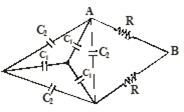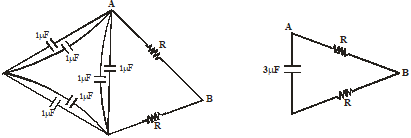Capacitance
Question

# In circuit diagram capacitance of capacitor ${\mathrm{C}}_{1}=3\mu \mathrm{F}$ and ${\mathrm{C}}_{2}=1\mu \mathrm{F}$. It is given that time constant of circuit between A and B is 3 millisecond. Value of R will beDifficult
Solution

## Equivalent circuit can be drawn as below$\begin{array}{l}\mathrm{RC}=3×{10}^{-1}\mathrm{sec}\\ \mathrm{R}×3×{10}^{-6}=3×{10}^{-3}\\ \mathrm{R}=1000\mathrm{\Omega }\end{array}$$\therefore$  Correct answer is (4)

Get Instant Solutions